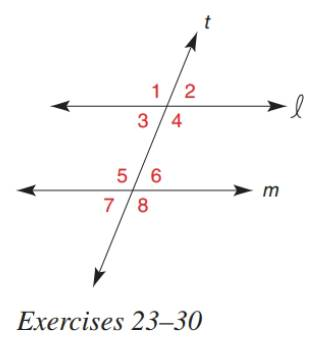Chapter 2.3, Problem 30E### Elementary Geometry for College St...

6th Edition
Daniel C. Alexander + 1 other
ISBN: 9781285195698

#### Solutions

Chapter
Section### Elementary Geometry for College St...

6th Edition
Daniel C. Alexander + 1 other
ISBN: 9781285195698
Textbook Problem
1 views

# In Exercise 23 to 30, determine the value of x so that line l will be parallel to line m . m ∠ 2 = ( x 2 − 1 ) ( x + 1 ) m ∠ 8 = 185 − x 2 ( x + 1 )To determine

To find:

The value of x for the given statement.

Explanation

Given:

The given statement is,

Line 𝓁 and m are cut by transversal t and are parallel to each other.

m2=(x21)(x+1) and m8=185x2(x+1).

Figure (1)

Theorem:

(1) If two parallel lines are cut by transversal, then the pairs of corresponding angles are congruent

(2) Angles on a straight line are supplementary.

Calculation:

Line 𝓁 is parallel to line m.

8 and 6 are are supplementary angles.

So,

m8+m6=180

Substitute 185x2(x+1) for m8 in the above equation.

185x2(x+1)+m6=180m6=180185+x2(x+1)m6=x2(x+1)5

2 and 6 are corresponding angles

### Still sussing out bartleby?

Check out a sample textbook solution.

See a sample solution

#### The Solution to Your Study Problems

Bartleby provides explanations to thousands of textbook problems written by our experts, many with advanced degrees!

Get Started

#### In Exercises 2340, find the indicated limit. 28. limt3(4t22t+1)

Applied Calculus for the Managerial, Life, and Social Sciences: A Brief Approach

#### Evaluate: (6+4)82+3

Elementary Technical Mathematics

#### Sketch the graphs of the equations in Exercises 512. xy=x2+1

Finite Mathematics and Applied Calculus (MindTap Course List)

#### limx1x3x21x = _____. a) 1 b) 1 c) 0 d) does not exist

Study Guide for Stewart's Single Variable Calculus: Early Transcendentals, 8th

#### The scalar projection of on is:

Study Guide for Stewart's Multivariable Calculus, 8th